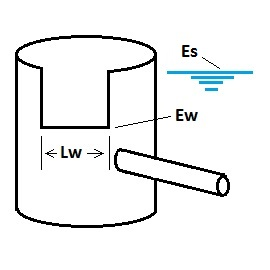* Log In to use the Calculate function * Become a Member!

Calculate Discharge From A Weir Outlet Control With Free Discharge:

Calculation:
Designer/Checker:

Input:(Note: All input Metric units are converted to English units for the equations below and then the output English units are converted back to Metric)

Only check the button below if applicable, otherwise leave unchecked.

Length of weir (Lw - ft or m)
Water surface elevation at design volume (Es - ft or m)
Elevation of crest of weir (Ew - ft or m)
Weir coefficient (Cw)

Typical values for Cw (English Units):
Rectangular weir - sharp crested: Cw = 3.33
Rectangular weir - broad crested: Cw = 2.861
Cipoletti trapezoid: Cw = 3.367
Ogee spillway: Cw = 3.97

Output: